﻿ Genetic Algorithm Based PID Parameter OptimizationAmerican Journal of Intelligent Systems

p-ISSN: 2165-8978    e-ISSN: 2165-8994

2020;  10(1): 8-13

doi:10.5923/j.ajis.20201001.02

Received: Nov. 17, 2020; Accepted: Nov. 29, 2020; Published: Dec. 15, 2020### Genetic Algorithm Based PID Parameter Optimization

Tanvir Ahmmed1, Irin Akhter1, S. M. Rezaul Karim1, F. A. Sabbir Ahamed2

Correspondence to: Tanvir Ahmmed, Department of EEE, International University of Business Agriculture and Technology, Bangladesh.
 Email: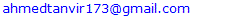Abstract

Knowing the value of PID parameters is important to tune the PID controller. There are different kinds of process to know the value of PID parameters. Genetic Algorithm is applied to find out the best value of PID parameters. Simulation process has been done by using code in MATLAB to initiate PID controller. In this work it has been shown that how to get the suitable value of PID parameters to tune the PID controller. Researching on different kinds of paper to get information about the process, at which we get the best value of PID parameters. The operation of PID controller depends on the value of PID Parameters. A block diagram shown the methodology part at which explain the whole process of this work. Performance Analysis of the procedure that applied to find the value of PID parameters. The value, which getting by using Genetic Algorithm is applied in (1st to 10th) order to calculate the fitness function. Different kinds of calculation, figure, graph and table shown in this work. After calculating the fitness value, it is obtained that which value is suitable for tuning the PID controller. These value is the resulting value to tune the PID controller.

Keywords: Genetic Algorithm, PID Controller, Calculation Fitness Function of PID Parameters, Applying Genetic Algorithm, Mat Lab work

Cite this paper: Tanvir Ahmmed, Irin Akhter, S. M. Rezaul Karim, F. A. Sabbir Ahamed, Genetic Algorithm Based PID Parameter Optimization, American Journal of Intelligent Systems, Vol. 10 No. 1, 2020, pp. 8-13. doi: 10.5923/j.ajis.20201001.02.

### 1. Introduction

The Value of PID parameters is important to tune the PID controller. There are several Methods to optimize the value of PID parameters those are metaheuristics, Trial and Error Method, Process reaction curve technique, Zeigler-Nichols method, MIMO process and other methods. Through this PID controller, can be controlled flow, temperature, speed etc. To control these things, the value of PID parameters is necessary. According to Tom O’Mahony, C. J. Downing and Klaudiusz Fatla (1996) without knowing exact the value of PID parameters, its complex process to tune PID controllers. To reduce the error of these PID controller Genetic Algorithm is used . Mainly PID is used in Industrial Purpose so, according to S.M. Giriraj Kumar, R. Jain, N. Anantharaman, V. Dharmalingam and K.M.M. Sheriffa Begum Genetic Algorithm is used to tune PID controller for improving the performance of bioreactor . In Genetic Algorithm, there are several components those are initial population, fitness function, select values, cross over and mutation are used to get best value. As these functions are working together in Genetic Algorithm process to find a value so, it is possible to get less error in the output value. Also another process is Zeigler-Nichols method according to T. Yucelen, O. Kaymakci and S. Kurtulan it is possible to find the value for PID parameter to tune the PID controller but in large overshooting purpose this method doesn’t work properly . By using Genetic Algorithm, it is possible to find the best value for PID controller as well as large overshooting Purposes.

### 2. PID Controller

PID is an acronym. Full form of PID controller is Proportional, Integral and Derivative. These three controllers are together make a control signal. Where Proportional improves the rise time, integral reduce the steady state error and derivative reduce the overshoot. PID is a feedback control system where PID controllers use a control loop feedback mechanism to control process variables. Which are Kp, Ki and Kd.

#### 2.1. Components of PID Parameters

PID parameters have three components which are Proportional, Integral and Derivative. Each of them has their own objective. Like Proportional improves the rise time, integral reduce the steady state error and derivative reduce the overshoot. These three controllers are together make a control signal. These three types of controllers can be combined into new controllers, which are given below :
1. Proportional and integral controllers (PI Controller)
2. Proportional and derivative controllers (PD Controller)
3. Proportional integral derivative control (PID Controller)
Adjust each of these three controllers Kp (Proportional), Ki (Integral) and Kd (Derivative) until obtain an aimed value or result. But in a single a system does not need to apply all these three (Proportional, Integral and Derivative) controllers, if it is not necessary. If in a system applying one controller like proportional controller gives the aimed value or result than others controllers are not required. In PID controller all these components are very important because each of them have their own purpose. In a feedback system PID controller maintains the output to the process [2,4,7]. If error signal with respect to time than Proportional, Integral and Derivative remain constant.

#### 2.2. PI Controller

Digital controller’s square measure enforced with distinct sampling periods and a distinct variety of the PI equation is required to approximate the integral of the error. This modification replaces the continual variety of the integral with a summation of the error and uses Δt because the time between sampling instances and nt because the range of sampling instances.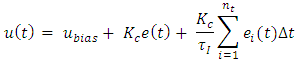PI management is required for non-integrating processes that means any method that eventually returns to identical output given identical set of inputs and disturbances. A P-only controller is best suited to group action processes. Integral action is employed to get rid of offset and might be thought of as associate degree adjustable ubias.

#### 2.3. PD Controller

A proportional-derivative (PD) controller may be wont to create a straightforward system track some point of reference. The suspension Associate in nursing exceedingly automobile is an analogue example: the spring and damper work along the car at some desired height. The spring exerts a force proportional its deflection, whereas the damper opposes motion (the spinoff of deflection).
A Pd controller uses an equivalent principles to form a virtual spring and damper between the measured and reference positions of a system. Higher than is associate in Nursing example showing a simulated point-mass (blue dot) that's trailing a target (green circle). Attempt clicking or dragging to maneuver the target around.
The response of a Pd controller may be characterized by 2 numbers: the damping quantitative relation and also the natural frequency. If the damping quantitative relation is a smaller amount than one, then the system can bit by bit approach the target. If the damping quantitative relation is larger than one, the system can shoot past the target before returning. The natural frequency describes however quickly the system approaches the target.

### 3. Genetic Algorithm

Genetic algorithm is a method to find the best value for any kind of optimization like constrained and unconstrained optimization problem by the process of natural selection that has a biological evolution.

#### 3.1. Types of Components in Genetic Algorithm

There are some genetic components that mainly used in Genetic Algorithm to guide the Algorithm process to give a best solution for a given problem. Genetic Algorithm have five components those are [9,10]:
Ø Initial population
Ø Fitness function
Ø Selection
Ø Crossover
Ø Mutation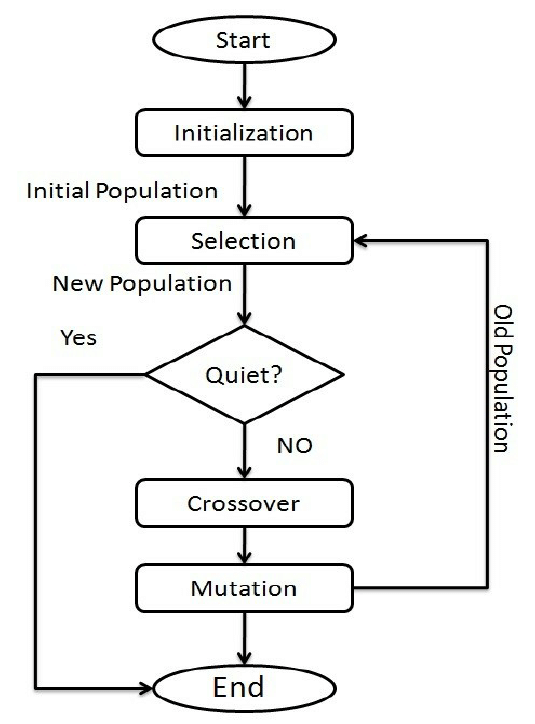Figure 1. Flowchart of Genetic Algorithm
There are three main components those components are selection, crossover and mutation. These components are work together so that the algorithm work properly.

### 4. Ziegler- Nichols Model

Thus the Ziegler-Nichols model assumes an additional hypothetical stage combination that does not distort the Rolf of the supposed size. At the margin of stability, there is a 180-degree phase shift around the feedback loop. The first order lag cannot contribute more than 90 degrees to that phase shift. The rest of the observed phase shift must be covered by a combination of artificial episodes. Phase adjustment is considered to be a straight line between zero and critical frequencies where a 180-degree episode shift occurs. A “straight line” phase shift corresponds to an actual time delay.

### 5. Literatures Review

The following are some of the books and papers mentioned below the process of accepting this project. To accept his project, the genetic algorithm needs to be thoroughly read before the project can be completed the beginning. Thus a comprehensive study of resources is required and below is some literature that somehow contributes my understanding of control systems and genetic algorithms specific.
G.A. searching for the best global solution to a problem is not guaranteed, but they are usually very good at finding acceptable solutions to the problem. Acceptably fast where there is special technology to solve special problem, they can perform both speed and GAs the accuracy of the final result, so there is no magic in evolution the calculation should therefore use GAs when no other unknown efficient problem solving strategies.
To initiate the value of PID parameter metaheuristics, Trial and Error Method, Process reaction curve technique, Zeigler-Nichols method, MIMO process and other process is used. Through PID controller temperature, speed, flow etc. can be controlled. The rules of tuning work quite effectively when you have an analog controller, a system that is linear, monotonous and slow, and a response that uses a single-pole intimate "leg" or something that is affected by [19,20]. The actual plants are less likely to have a perfect first-order back feature, but in most cases this estimate is reasonable to describe the frequency response Rolf. Higher order poles, however, will introduce an additional step shift. Even if they don’t affect the size of the gain rollover too much, the phase shift is much more important for stability. You cannot rely on a single "leg" pole to match both the amplitude roll-off and the phase shift.
Thus the Ziegler-Nichols model assumes an additional hypothetical stage combination that does not distort the Rolf of the supposed size. At the margin of stability, there is a 180-degree phase shift around the feedback loop. The first order lag cannot contribute more than 90 degrees to that phase shift. The rest of the observed phase shift must be covered by a combination of artificial episodes. Phase adjustment is considered to be a straight line between zero and critical frequencies where a 180-degree episode shift occurs. A “straight line” phase shift corresponds to an actual time delay.
In short, the Ziegler-Nichols rule assumes that the following form of the system has a transfer function:The model matches the response of the system to the range of frequency 0 and stability and everything in between is more or less created.
To control the heater temperature, PID controller is used by the process of Genetic Algorithm , is a process to get the exact value of those parameter to tune the PID controller.
Without knowing exact the value of PID parameters, its complex process to tune PID controllers. To reduce the error of these PID controller Genetic Algorithm is used .
Optimizing the value of PID parameter is an important problem. To optimize the value of PID parameter Genetic Algorithm used which have lots of improvements like population, selection, crossover and mutation. The result of simulation Shows that the PID controller have better performance .
A nonlinear PID controller varies its parameter on time because of Output result. To get accurate value without error even in short time Genetic Algorithm, PID controller is used .
The Objective function of Optimization are integral of overshoot, rise time, absolute error and controller output. To optimize the value of PID parameters Genetic Algorithm is applied and Simulation results represent the effective method that is Genetic Algorithm .
In this project, GA is still being used as a predefined customized for tuning PID controller. You will see it some other optimization methods may be better in certain cases applications and GA One more application might be better. So no quick and fast rules that need to use optimization methods. It depends complexity of application and optimized implementation Algorithm.

### 6. Research Methodology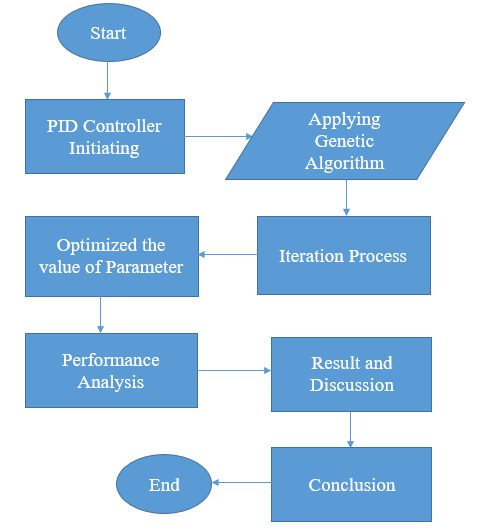Figure 2. Procedure of the Research Work

#### 6.1. Applying Genetic Algorithm

There are some genetic components that are used in Genetic Algorithm to guide the Algorithm process to give a best solution for a given problem. The genetic algorithm as an intelligent method has also been widely used to tune the parameters of PID [5,6]. Genetic algorithms are used to create an objective function that evaluate the PID gains based on the overall error of the systems and generate high quality solution.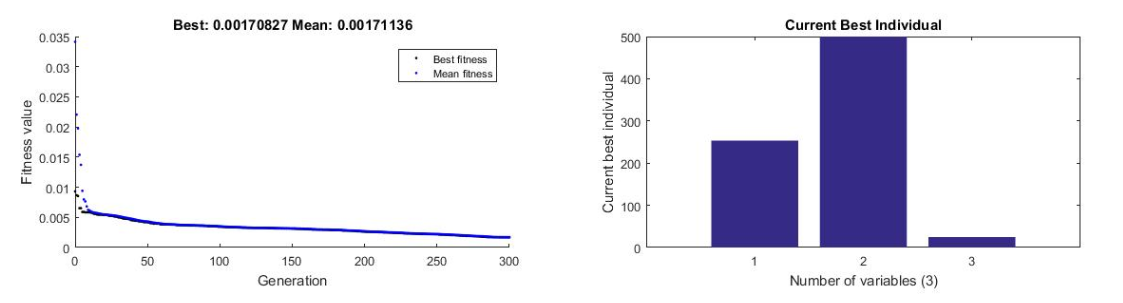Figure 3. Best Fitness Mean & Current Individuals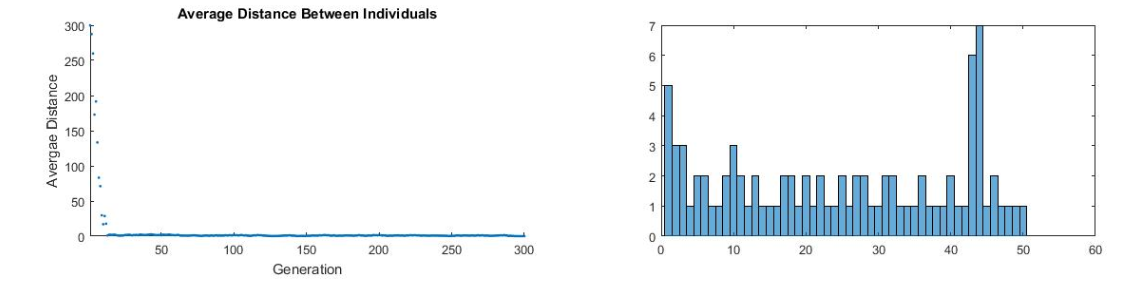Figure 4. Average Distance between Individuals

### 7. Result and Discussion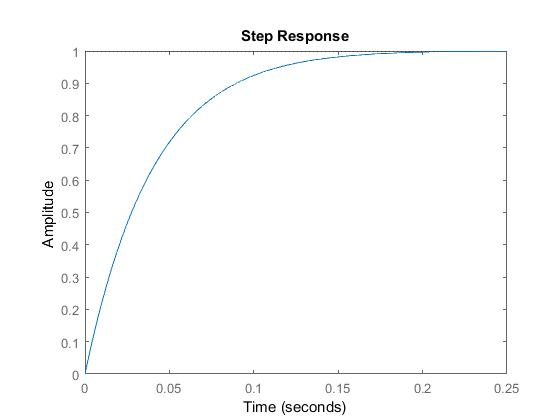Figure 5. Tuning PID Controller

#### 7.1. Comparison among Different Order Fitness Function

 Table 1. Fitness Function of different orders PID Parameters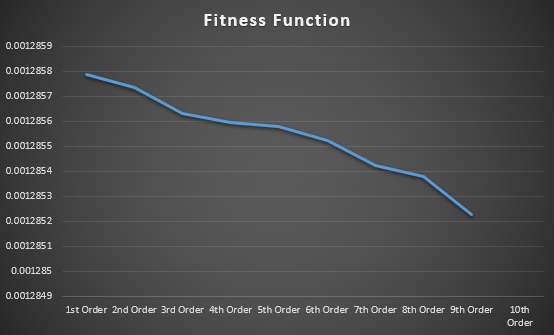Figure 6. Line Chart of Fitness Function for Different Orders of PID Parameters
Above this line chat and table, we shown the value of Fitness function in different orders. From this value we may understand that the value we are getting from fitness calculation which are higher to lower that are responsible for tuning the PID controller.

### 8. Conclusions

PID controller is very useful for industrial control applications to manage flow, temperature, speed and many other variables. So PID controllers need to give accurate value without any error. In this purpose we can use Genetic Algorithm in PID controllers to give accurate value within less time.
In this report chapter 2, showed the theoretical overview of PID parameters and Genetic Algorithm. Here we can see the parts of PID parameters and Genetic Algorithm [8,11,12].
Genetic Algorithm is applied to find out the best value of PID parameters. Using MATLAB we can see difference between Genetic Algorithm based PID controller and without Genetic Algorithm based PID controller. In this report chapter 4, showed that how genetic Algorithm used in PID controllers and gives accurate value. Here graph shows that different points have different value. Without Genetic Algorithm PID controller shows a different graph.
Fitness function is one part of Genetic Algorithm. It is also helps us to see difference between the value. It helps to identify that which value is suitable for PID controller. In chapter 5, showed that the calculation and comparison different orders of Fitness function [15,16].
Mainly, PID controller use in Industrial purposes so the value should be accurate. Applying Genetic Algorithm is easy to get accurate or best value in PID controller. So after doing this report we can see that Genetic Algorithm based PID controller is more easy and useful [13,14,17].

### References

  Dr Vipin Jain October 11, 2020. Types of Controllers | Proportional Integral and Derivative Controllers. Researchgate.  SAIFUDIN BIN MOHAMED IBRAHIM 27th October, 2005. THE PID CONTROLLER DESIGN USING GENETIC ALGORITHM. Researchgate.  Kiam Heong Ang; G. Chong; Yun Li 20 June 2005. PID control system analysis, design, and technology. Researchgate.  Bhawna Tandon August 2011. Genetic algorithm based parameter tuning of PID controller for composition control system. Researchgate.  Dwi ANA Ratna Wati, Rakhmat Hidayat November 2013. Genetic algorithm-based PID parameters optimization for air heater temperature control. Researchgate.  D. C. Meena; Ambrish Devanshu 16 October 2017. Genetic algorithm tuned PID controller for process control. Coimbatore, India: IEEE Press.  T O.Mahony, C J Downing and K Fatla, 2020 “Genetic Algorithm for PID Parameter Optimization: Minimizing Error Criteria”, Process Control and Instrumentation 2000 26-28 July 2000, University of Stracthclyde, pg 148-153, July 2000.  A. Varsek, T. Urbacic and B. Filipic, 1993 “Genetic Algorithms in Controller Design and Tuning”, IEEE Trans.  Samakwong T. and Assawinchaichote W., 2-4 March 2016. PID Controller Design for Electro-Hydraulic Servo Valve System with Genetic Algorithm. Proc International Electrical Engineering Conress, ChiangMai, Thailand.  Mitchell Melanie 1999. An Introduction to Genetic Algorithms (Book). Cambridge, Massachusetts • London, England: MIT Press.  Bhawna Tandon, Randeep Kaur 2011. Genetic Algorithm Based Parameter Tuning of Pid Controller for Composition Control System. Mullana: Researchgate.  J Zhao, M Xi - MS&E, 2020. Self-Tuning of PID Parameters Based on Adaptive Genetic Algorithm. Jimin Zhao and Miao: iopscience press.  K.R. Uren* and G. van Schoor* 2012. Genetic Algorithm based PID Tuning for Optimal Power Control of a Threeshaft Brayton Cycle based Power Conversion Unit. South Africa: IEEE Press.  Yuqi Fan, Junpeng Shao,* and Guitao Sun 2019. Optimized PID Controller Based on Beetle Antennae Search Algorithm for Electro-Hydraulic Position Servo Control System. China: Sensors (Basel).  Yuqi Fan, Junpeng Shao, Guitao Sun and Xuan Shao 17 January 2020. Proportional–Integral–Derivative Controller Design Using an Advanced Lévy-Flight Salp Swarm Algorithm for Hydraulic Systems. China: Researchgate.  Sandeep Bhongade*1, Barjeev Tyagi*2, H. O. Gupta*3 2011. Genetic algorithm based PID controller design for a multi-area AGC scheme in a restructured power system. India: Researchgate.  Dwi Ana Ratna Wati; Rakhmat Hidayat 2013. Genetic algorithm-based PID parameters optimization for air heater temperature control. Jogjakarta, Indonesia: IEEE Press.  Dwi Ana Ratna Wati and Rakhmat Hidayat 2013. Genetic algorithm-based PID parameters optimization for air heater temperature control. Yogyakarta, Indonesia: IEEE Press.  Tom O’Mahony, C. J. Downing and Klaudiusz Fatla 1996. Genetic Algorithms for PID Parameter Optimisation: Minimising Error Criteria. Cork, IRELAND: Researchgate.  Xiangzhong Meng and Baoye Song 2007. Fast Genetic Algorithms Used for PID Parameter Optimization. Jinan, China: IEEE Press.  Mehmet Korkmaz, Ömer Aydoğdu, Hüseyin Doğan 2012. Design and Performance Comparison of Variable Parameter Nonlinear PID Controller and Genetic Algorithm Based PID Controller. Konya-Turkey: IEEE Press.  Hong Zhang, Yuanhu Cai, Yuchun Chen 2010. Parameter optimization of PID controllers based on genetic algorithm. Shenzhen, China: IEEE Press.